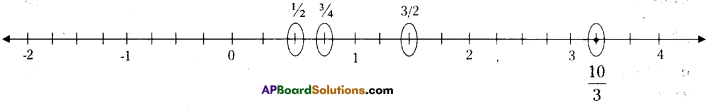# AP Board 7th Class Maths Solutions Chapter 2 Fractions, Decimals and Rational Numbers InText Questions

AP State Syllabus AP Board 7th Class Maths Solutions Chapter 2 Fractions, Decimals and Rational Numbers InText Questions and Answers.

## AP State Syllabus 7th Class Maths Solutions 2nd Lesson Fractions, Decimals and Rational Numbers InText QuestionsDo This

Question 1.
Write five examples, each of proper, improper, mixed fractions. ? (Page No. 27)
Solution:
Proper fractions
$$\frac{1}{5}, \frac{2}{3}, \frac{4}{7}, \frac{3}{8}, \frac{4}{9}$$

Improper fractions
$$\frac{7}{2}, \frac{3}{2}, \frac{9}{4}, \frac{11}{5}, \frac{8}{3}$$

Mixed fractions
$$1 \frac{2}{3}, 2 \frac{3}{5}, 4 \frac{1}{7}, 8 \frac{6}{7}$$Question 2.
Write five equivalent fractions for. i) $$\frac{3}{5}$$ ii) $$\frac{4}{7}$$ (Page No. 28)
Solution: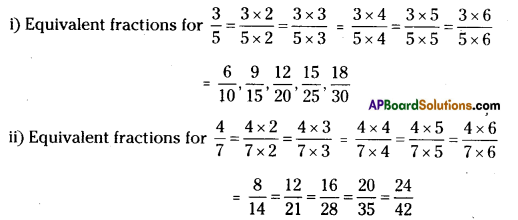Do This

Question 1. (Page No. 31)
i) 4 x $$\frac { 2 }{ 7 }$$
ii) 4 x $$\frac { 3 }{ 5 }$$
iii) 7 x $$\frac { 1 }{ 3 }$$
Solution:
i) 4 x $$\frac{2}{7}=\frac{4 \times 2}{7}=\frac{8}{7}$$
ii) 4 x $$\frac{3}{5}=\frac{4 \times 3}{5}=\frac{12}{5}$$
iii) 7 x $$\frac{1}{3}=\frac{7 \times 1}{3}=\frac{7}{3}$$

Question 2.
Find i) 5 x $$\frac { 3 }{ 2 }$$ =
ii) 4 x $$\frac { 7 }{ 5 }$$ =
iii) 7 x $$\frac { 8 }{ 3 }$$ = (Page No. 31)
Solution:
i) 5 x $$\frac{3}{2}=\frac{5 \times 3}{2}=\frac{15}{2}=7 \frac{1}{2}$$
ii) 4 x $$\frac{7}{5}=\frac{4 \times 7}{5}=\frac{28}{5}=5 \frac{3}{5}$$
iii) 7 x $$\frac{8}{3}=\frac{7 \times 8}{3}=\frac{56}{3}=18 \frac{2}{3}$$Question 3.
Find the following: (Page No. 32)
i) 3 x 2 $$\frac{2}{7}$$
ii) 5 x 2$$\frac{1}{3}$$
iii) 8 x 4$$\frac{1}{7}$$
iv) 4 x 1$$\frac{2}{9}$$
v) 5 x 1$$\frac{1}{3}$$
Solution: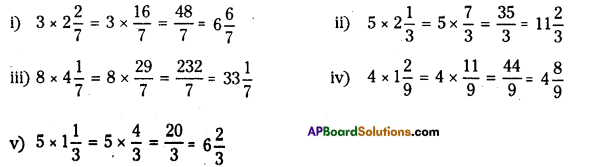Do These

Question 1.
Fill in these boxes. (Page No. 35)
i) $$\frac{1}{5} \times \frac{1}{7}=\frac{1 \times 1}{5 \times 7}$$ = ……………
ii) $$\frac{1}{2} \times \frac{1}{6}=\frac{1 \times 1}{2 \times 6}=$$ = …………………
Solution:
i) $$\frac{1}{5} \times \frac{1}{7}=\frac{1 \times 1}{5 \times 7}=\frac{1}{35}$$
ii) $$\frac{1}{2} \times \frac{1}{6}=\frac{1 \times 1}{2 \times 6}=\frac{1}{12}$$

Do This

Question 1.
Find (Page No. 39)
i) 2 ÷ $$\frac{1}{4}$$
ii) 7 ÷ $$\frac{1}{2}$$
iii) 3 ÷ $$\frac{1}{5}$$ (Page No. 39)
Solution:
i) 2 ÷ $$\frac{1}{4}=2 \times \frac{4}{1}=\frac{8}{1}$$ = 8
ii) 7 ÷ $$\frac{1}{2}=7 \times \frac{2}{1}$$ = 14
iii) 3 ÷ $$\frac{1}{5}=3 \times \frac{5}{1}$$ = 15

Question 2.
Find (Page No. 41)
i) 9 ÷ $$\frac{2}{5}$$
ii) 3 ÷ $$\frac{4}{7}$$
iii) 2 ÷ $$\frac{8}{9}$$
Solution:
i) 9 ÷ $$\frac{2}{5}$$ = $$9 \times \frac{5}{2}=\frac{45}{2}$$
ii) 3 ÷ $$\frac{4}{7}$$ = $$3 \times \frac{7}{4}=\frac{21}{4}$$
iii) 2 ÷ $$\frac{8}{9}$$ = $$2 \times \frac{9}{8}=\frac{9}{4}$$Question 3.
Find (Page No. 41)
i) 7 ÷ 5$$\frac{1}{3}$$
ii) 5 ÷ 2$$\frac{4}{7}$$
Solution:
i) 7 ÷ 5$$\frac{1}{3}$$ = $$7 \div \frac{16}{3}=7 \times \frac{3}{16}=\frac{21}{16}$$
ii) 5 ÷ 2$$\frac{4}{7}$$ = $$5 \div \frac{18}{7}=5 \times \frac{7}{18}=\frac{35}{18}$$

Question 4.
Find (Page No. 42)
i) $$\frac{3}{5} \div \frac{1}{2}$$
ii) $$\frac{1}{2} \div \frac{3}{5}$$
iii) $$2 \frac{1}{2} \div \frac{3}{5}$$
iv) $$5 \frac{1}{6} \div \frac{9}{2}$$
Solution: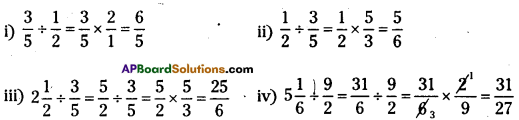Do This

Question
Find (Page No. 45)
i) 0.25 + 5.30
ii) 29.75 – 25.97
Solution:
i) 0.25 + 5.30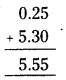ii) 29.75 – 25.97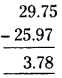Do These

Question 1.
Find (Page No. 48)
i) 1.7 x 3
ii)2.0 x 1.5
iii) 2.3 x 4.35
Solution:
i)1.7 x 3 = 5.1
ii) 2.0 x 1.5 = 3.00
iii) 2.3 x 4.35 = 10.005

Question 2.
Arrange the products obtained in (I) In descending order.
Solution:
Arranging above answers in descending order 10.005 > 5.1 > 3.00

Question 3.
Find (Page No. 50)
i) 35.7 ÷ 3
ii) 25.5 ÷ 3
Solution: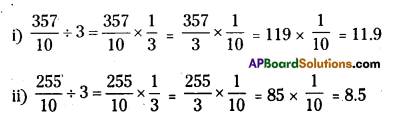Do These

Question 1.
Find the greatest and the smallest numbers among the following groups. (Page No. 52)
i) 2, -2, -3, 4, 0, -5
ii) -3, -7, -8,0,-5,-2
Solution:
i) 2, -2, -3, 4, 0, -5 : greatest number = 4; smallest number = -5
ii) -3, -7, -8, 0, -5, -2: greatest number = 0; smallest number = -8Question 2.
Write the following numbers In ascending order. (Page No. 52)
i) -5,-75,3,-2,4, $$\frac{3}{2}$$
ii) $$\frac{2}{3}, \frac{3}{2}$$, 0, -1, -2, 5
Solution:
i) -5, -75, 3, -2, 4, $$\frac{3}{2}$$
Ascending order = -75 <-5 <-2 < $$\frac{3}{2}$$ <3 < 4 or -75, -5, -2, $$\frac{3}{2}$$, 3, 4

ii) $$\frac{2}{3}$$,$$\frac{3}{2}$$, 0, -1, -2, 5
Ascending order = -2, -1, 0, $$\frac{2}{3}$$, $$\frac{3}{2}$$, 5

Question 3.
Write 5 equlvalent rational numbers to (i) $$\frac{5}{2}$$ (Page No. 56)
(ii) $$\frac{-7}{8}$$
(iii) $$\frac{-3}{7}$$
Solution: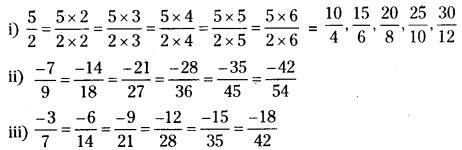Do These

Question 1.
Which is bigger $$\frac{5}{8}$$ or $$\frac{3}{5}$$ ? (PageNo.28)
Solution:
$$\frac{5}{8} \times \frac{5}{5}=\frac{25}{40}$$ and $$\frac{3}{5} \times \frac{8}{8}=\frac{24}{40}$$
As $$\frac{24}{40}<\frac{25}{40}$$ $$\frac{5}{8}$$ is bigger than $$\frac{3}{5}$$

Question 2.
Determine if the following pairs are equal by writing each in their simplest form. (Page No. 28)
i) $$\frac{3}{8}$$ and $$\frac{375}{1000}$$
Solution: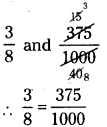ii) $$\frac{18}{54}$$ and $$\frac{23}{69}$$
Solution: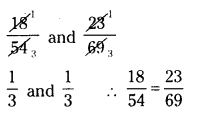iii) $$\frac{6}{10}$$ and $$\frac{600}{1000}$$
Solution: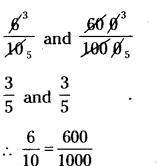iv) $$\frac{17}{27}$$ and $$\frac{25}{45}$$
Solution”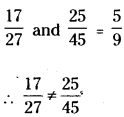Do These

Question 1.
Identify the equivalent rational number is each question
i) $$\frac{-1}{2}, \frac{-3}{4}, \frac{-2}{4}, \frac{-4}{8}$$
Solution:
$$\frac{-1}{2}=\frac{-2}{4}=\frac{-4}{8}$$

ii) $$\frac{1}{4}, \frac{3}{4}, \frac{5}{3}, \frac{10}{6}, \frac{2}{4}, \frac{20}{12}$$
Solution:
$$\frac{5}{3}=\frac{20}{12}=\frac{10}{6}$$Try This

Question 1.
You have seen that the product of two natural numbers is one or more than one is bigger than each of the two natural numbers. For example 3 x 4 = 12; 12 > 4 and 12 > 3. What happens to the value of the product when we multiply two proper fractions? (Page No.37)
Fill the following table and conclude your observations.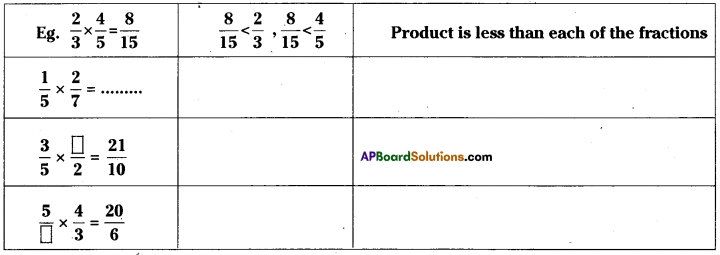Solution: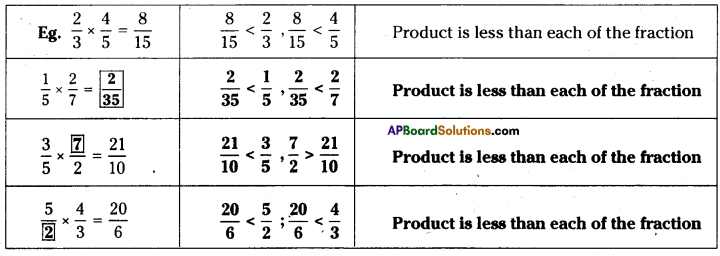Question 2.
Will the reciprocal of a proper fraction be a proper fraction? (Page No. 40)
Solution:
No. Reciprocal of a proper fraction is always an improper fraction.

Question 3.
Will the reciprocal of an Improper fraction be an Improper fraction?
Solution:
No. The reciprocal of an improper fraction is always a proper fraction.Question 4.
Look at the following table and fill up the blank spaces. (Page No. 44)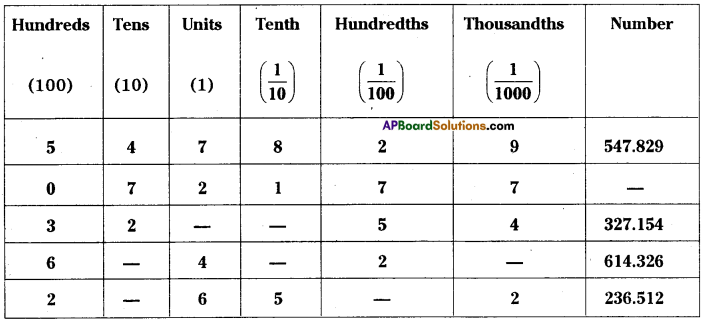Solution: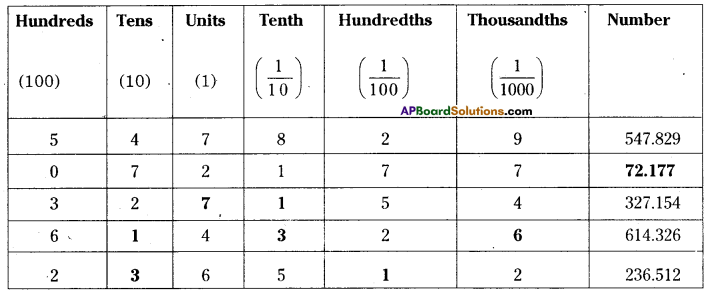Question 5.
WrIte the following numbers in their expanded form. (Page No.44)
i) 30.807
ii) 968.038
iii) 8370. 705
Solution:
i) 30.8O7 = 10 x 3 + 1 x 0 + $$\frac{1}{10}$$ x 8 +$$\frac{1}{100}$$ x 0 + $$\frac{1}{1000}$$ x 7 = 30 + $$\frac{8}{10}+\frac{7}{1000}$$

ii) 968.038 = 100 x 9 + 10 x 6 + 1 x 8 + $$\frac{1}{10}$$ x 0 + $$\frac{1}{100}$$ x 3 + $$\frac{1}{1000}$$ x 8 = 900 + 60 + 8 + $$\frac{3}{100}+\frac{8}{1000}$$

iii) 8370.705 = 1000 x 8 + 100 x 3 + 10 x 7 + $$\frac{1}{10}$$ x 7 + $$\frac{1}{1000}$$ x 5 = 8000 + 300 + 70 + $$\frac{7}{10}+\frac{5}{1000}$$Question 6.
Take any5 Integers and make all possible rational numbrs with them. (Page No. 54)
Solution:
Consider 2, 3, 4, 5 and 7
Ratlonalnumberare $$\frac{2}{3}, \frac{2}{4}, \frac{2}{5}, \frac{2}{7}, \frac{3}{4}, \frac{3}{5}, \frac{3}{7}, \frac{3}{2}, \frac{4}{2}, \frac{4}{3}, \frac{4}{5}, \frac{4}{7}, \frac{5}{2}, \frac{5}{3}, \frac{5}{4}, \frac{5}{7}, \frac{7}{2}, \frac{7}{3}, \frac{7}{4}, \frac{7}{5}$$

Question 7.
Consider any 5 rational numbers. Find out which itegers constitute them? (Page No.54)
Solution:
Take $$\frac{3}{4}, \frac{5}{8}, \frac{6}{11}, \frac{2}{7}$$ and $$\frac{1}{5}$$.
The integers are 1, 2, 3, 4, 5, 6, 7, 8 and 11.

Do This

Question 1.
Find (i) 50 paise = ₹………….. (ii) 22 g = ………….. kg (iii) 80 cm = ………………m (Page No. 44)
Solution:
(i) 50 paise =₹$$\frac{50}{100}$$ = ₹ 0.5
(ii) 22 g = $$\frac{22}{1000}$$ kg = 0.022 kg
(iii) 80 cm = $$\frac{80}{100}$$ m = 0.8 mTry This

Question 1.
Represent $$\frac{3}{4}$$ and $$\frac{1}{4}$$ in different ways using different figures. Justify your representation. Share, and check it with your friends. (Page No.27)
Solution: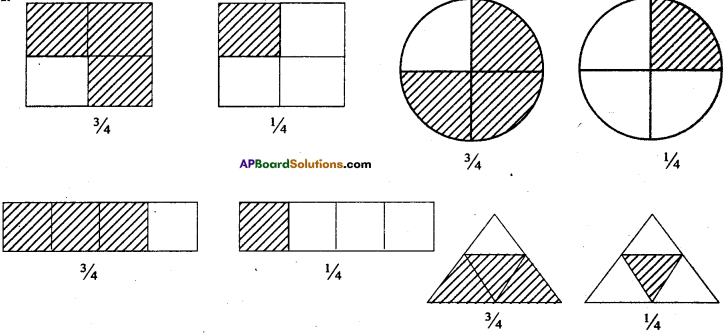Question 2.
Represents 2 1/4 pictorially. How many units are needed for this. (Page No. 27)
Solution: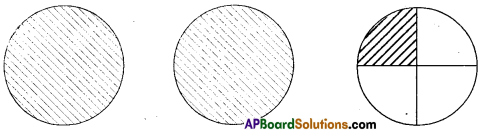We need 3 units to represent 2½

Do These

Question 1.
Represent pictorially 2 x $$\frac{1}{5}=\frac{2}{5}$$ (Page No. 32)
Solution: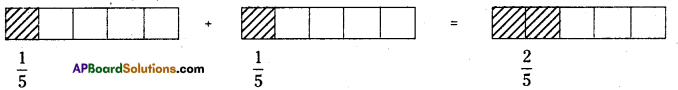Do These

Question 1.
Find $$\frac{1}{2} \times \frac{1}{5}$$ and $$\frac{1}{5} \times \frac{1}{2}$$ using diagram check whether $$\frac{1}{2} \times \frac{1}{5}=\frac{1}{5} \times \frac{1}{2}$$ (Page No. 35)
Solution: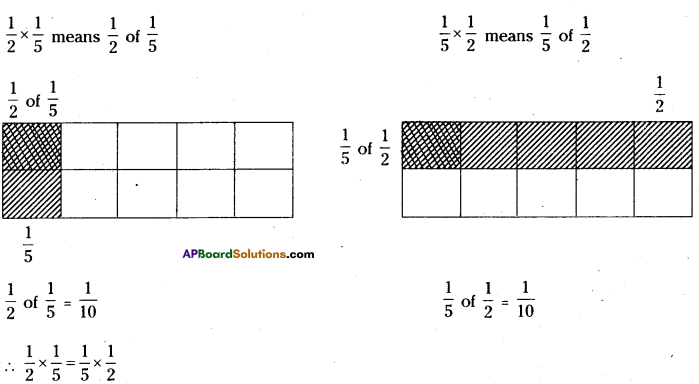Do These

Question 1.
Write 5 more fractions between (i) 0 and 1 (ii) 1 and 2. (Page No. 52)
Solution:
i) Fractions between 0 and 1 are $$\frac{1}{7}, \frac{2}{7}, \frac{3}{7}, \frac{4}{7}, \frac{5}{7}, \frac{6}{7}$$
ii) Fractions between land 2 are $$\frac{8}{7}, \frac{9}{7}, \frac{10}{7}, \frac{11}{7}, \frac{12}{7}, \frac{13}{7}$$

Question 2.
Where does 4$$\frac{3}{5}$$ lie on the number line? (Page No. 52)
Solution:
4$$\frac{3}{5}$$ lies between 4 and 5 on the number line.

Question 3.
On the number line given below represent the following numbei. (Page No.53)
i) $$\frac{-7}{2}$$
ii) $$\frac{3}{2}$$
iii) $$\frac{7}{4}$$
iv) $$\frac{-7}{4}$$
v) $$\frac{-1}{2}$$
vi) $$\frac{1}{4}$$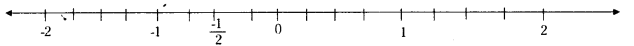Solution: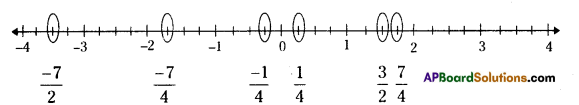Question 4.
Consider the following numbers on a number line. (Page No. 53)
27, $$\frac{-7}{8}, \frac{11}{943}, \frac{54}{17}$$, -68, -3, $$\frac{-9}{6}, \frac{7}{2}$$
i) Which of these are to the left of a) 0
Solution:
Left to zero are negative numbers
∴ $$\frac{-7}{8}$$, -68, -3, $$\frac{-9}{6}$$b) -2
Left to – 2 are less than – 2.
∴ -3, -68

c) 4
Left to 4 are less than 4.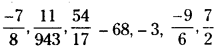d) 2
Left to 2 are less than 2.
∴ $$\frac{-7}{8}, \frac{11}{943}-68,-3, \frac{-9}{6}$$

ii) Which of these would be to the right of
a) 0
Right to zero are positive number.
∴ $$27, \frac{11}{943}, \frac{54}{17}, \frac{7}{2}$$

b) -5
Right to -5 are greater than -5.
$$27, \frac{-7}{8}, \frac{11}{943}, \frac{54}{17}-3, \frac{-9}{6}, \frac{7}{2}$$

c) 3$$\frac{1}{2}$$
Right to 3$$\frac{1}{2}$$ are more than 3$$\frac{1}{2}$$.
∴ 27

d) $$\frac{-5}{2}$$
Right to $$\frac{-5}{2}$$ are more than $$\frac{-5}{2}$$
∴ $$-27, \frac{-7}{8}, \frac{11}{943}, \frac{54}{17} \frac{-9}{6}, \frac{7}{2}$$Try These

Question 1.
Write three more equivalent fractions of $$\frac{3}{4}$$ and mark them on the number line. What do you observe? (Page No. 55)
Solution:
Equivalent fractions of $$\frac{3}{4}$$ lie on the same mark.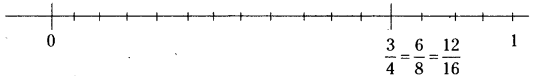Question 2.
Do all equivalent fractions of $$\frac{6}{7}$$ represent the same point on the number line.
(Page No. 55)
Solution:
Yes.

Question 3.
Are $$\frac{-1}{2}$$ and $$\frac{-3}{6}$$ represent same point on the number line? (Page No. 55)
Solution:
Yes.Question 4.
Are $$\frac{-2}{3}$$ and $$\frac{-4}{6}$$ equivalent? . (Page No. 55)
Solution:
Yes.

Question 5.
Mark the following rational numbers on the number line. (In Ex 7,3)
(i) $$\frac{1}{2}$$
(ii) $$\frac{3}{4}$$
(iii) $$\frac{3}{2}$$
(iv) $$\frac{10}{3}$$
Solution: